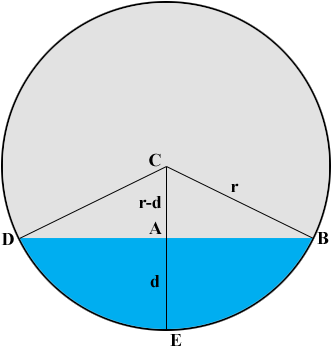SEARCH HOMEMath Central Quandaries & QueriesQuestion from Chip: Hello, I have a problem to which I know there must be an analytical solution - but as it has been 50 years since I studied math I can't remember quite how to do it!! I have a 12" round culvert in my yard that runs water all year. I would like to be able to calculate the flow through the culvert by measuring the depth of water flowing through and measuring the speed of the current. I can measure the depth and the speed, but I forget how to calculate the cross section of the flow. If you could help I would greatly appreciate it. Thank youHi Chip,You know r and d in the diagram. I'm going to calculate the area of the cross section of the water as the area of the sector CDEB minus the area of the triangle CDB.

Triangle CAB is a right triangle so useing Pythagoras theorem

(r - d)2 + |AB|2 = r2.

Solving for |AB| gives

|AB| = √(2rd - d2).

Now you can calculate the area of the triangle CDB using the fact that the area is one half the base times the height.

Looking at triangle CAB again I see that cos(angle BCA) = (r - d)/r and hence the measure of angle BCA is cos-1[(r-d)/r]. (When using your calculator to find cos-1 make sure it is set on degrees.) Hence the measure of the angle BCD is given by θ = 2 cos-1[(r-d)/r].

This angle is a fraction of one entire revolution of the circle, in fact it's θ/360 of the way around the circle. By the symmetry of the circle the area of the sector CDEB is θ/360 of the area of the circle. That is

area of the sector CDEB = (θ/360) × π r2.

I hope this helps,
PennyMath Central is supported by the University of Regina and The Pacific Institute for the Mathematical Sciences.